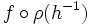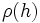# External wreath product

(Redirected from Wreath product)
This article describes a product notion for groups. See other related product notions for groups.

## Definition

### For an abstract group and a group of permutations

This definition uses the left action convention.

Let$G$ be any abstract group and$H$ be a group along with a homomorphism$\rho:H \to \operatorname{Sym}(S)$ for some set$S$ (in other words, we are given a permutation representation, or group action, of$H$). Then, the external wreath product of$G$ by$H$ (relative to$\rho$) is defined as the external semidirect product of the group$G^S$ by$H$, where an element$h \in H$ sends$f:S \to G$ to the function$f \circ \rho(h^{-1})$.

This wreath product is typically denoted as:$G \wr H$

The group$G$ is termed the base of the wreath product.

The wreath product can also be viewed as follows: The group$G^S$ is a direct product of$|S|$ copies of$G$, and the action of$h \in H$ is to permute the$|S|$ coordinates by the permutation$\rho(h)$.

There is a related notion of the restricted external wreath product. This is the same as above except that we replace functions$f:S \to G$ by finitely supported functions, i.e., functions for which the set of points for which the value is a non-identity element is finite.

### For two abstract groups

Let$G,H$ be abstract groups. Then, the external wreath product of$G$ by$H$ is typically understood as the wreath product where the action of$H$ is taken to be the regular group action on itself as a set. In other words, the homomorphism$\rho$ is the natural embedding arising via Cayley's theorem. This wreath product is also termed the regular wreath product.

### Equivalence with the internal wreath product

An internal wreath product is the equivalent to an external wreath product, except that here we start with the whole group, and find subgroups$G$ and$H$ such that the given group is an internal semidirect product of a direct product of copies of$G$ by$H$, acting via coordinate permutations only. A subgroup$G$ arising in this way is termed a base of a wreath product.

## Examples

Base Acting group Wreath product as an abstract group GAP ID
cyclic group:Z2 cyclic group:Z2 acting regularly dihedral group:D8 (8,3)
cyclic group:Z2 cyclic group:Z3 acting regularly direct product of A4 and Z2 (24,13)
cyclic group:Z2 symmetric group:S3 acting as a symmetric group direct product of S4 and Z2 (48,48)
cyclic group:Z3 cyclic group:Z2 acting regularly direct product of S3 and Z3 (18,3)
cyclic group:Z4 cyclic group:Z2 acting regularly wreath product of Z4 and Z2 (32,11)
group of prime order group of prime order (same prime, acting regularly) wreath product of groups of order p

## Facts

### The wreath product of two groups of permutations

Suppose both$G$ and$H$ are being viewed along with specific group actions of each, i.e., they are both being viewed as groups of permutations, with$G$ acting on a set$A$ and$H$ acting on a set$S$. Then,$G \wr H$ acts naturally on two sets: the Cartesian product$A \times S$, and the function space$A^S$ of functions from$S$ to$A$.

Further information: Action of wreath product on Cartesian product, Action of wreath product on function space

### Associativity of wreath product

The associativity law:$(G \wr H) \wr K \cong G \wr (H \wr K)$

holds with a suitable interpretation. For instance, if we are given the left side, we need to justify how$H \wr K$ is to be viewed as a group of permutations for the right side to make sense. Both$H$ and$K$ come as groups of permutations, and we equip$H \wr K$ with the induced action on the Cartesian product of the spaces they're acting on.

Further information: Wreath product is associative